• 1：sort，将这数字从小到大排列； 2：unique，将相邻且重复的数放到vector的尾部，然后返回指向第个重复元素的迭代器（需要注意的是，被放在尾部的数据有时会产生变化，所以不能继续使用了，需要废弃掉）； 3...
这个功能涉及到一个数据结构（vector）和三个函数：  1：sort，将这组数字从小到大排列；  2：unique，将相邻且重复的数放到vector的尾部，然后返回指向第一个重复元素的迭代器（需要注意的是，被放在尾部的数据有时会产生变化，所以不能继续使用了，需要废弃掉）；  3：erase，擦除重复的数据。  示例代码如下：
#include "stdafx.h"
#include <iostream>
#include <algorithm>
#include <vector>
using namespace std;

int main()
{
vector<int> v;
cout << "please input the number of vector's element" << endl;
int number;
cin >> number;
for (int i = 0; i < number; i++)
{
int temp;
cin >> temp;
v.push_back(temp);   //在vector尾部加入一个数据
}
sort(v.begin(),v.end());
v.erase(unique(v.begin(), v.end()), v.end());
for (int i = 0; i < v.size(); i++)
{
cout << v[i] << " ";
}
system("pause");
return 0;
}
展开全文函数
• 算法复杂度为O(n2n^{2}n2)，空间复杂度为O(111)。 1.1. 算法讲解 将第j个元素和后面的元素依次对比，如果大于序列为j+1的元素，就进行交换。 作为对比的基础元素j取值范围为"0 => size - 2"。因为j + 1不可...


目录
1. 冒泡排序1.1. 算法讲解1.2. 代码实现
2. 选择排序2.1. 算法讲解2.2. 代码实现
3 插入排序2.1. 算法讲解2.2. 代码实现
4 希尔排序2.1. 算法讲解2.2. 代码实现
5 归并排序2.1. 算法讲解2.2. 代码实现
6 快速排序6.1. 算法讲解6.2. 代码实现
7 堆排序2.1. 算法讲解2.2. 代码实现
8 计数排序2.1. 算法讲解2.2. 代码实现
9 桶排序2.1. 算法讲解2.2. 代码实现
10 基数排序2.1. 算法讲解2.2. 代码实现
附录1. 10大经典排序算法性能分析2. C/C++的内置排序函数2.1. c++的sort函数2.1.1. 函数简述2.1.2. cmp叙述2.1.3. 代码实现
2.2 c语言的qsort函数2.1.1. 函数简述2.1.2. cmp叙述2.1.3. 代码实现

1. 冒泡排序
冒泡排序，顾名思义，就是出头的被排序。其算法复杂度为O(

n

2

n^{2}

)，空间复杂度为O(

1

1

)。
1.1. 算法讲解
将第j个元素和后面的元素依次对比，如果大于序列为j+1的元素，就进行交换。作为对比的基础元素j取值范围为"0 => size - 2"。因为j + 1不可超过size - 1。因而，需要进行对比的j有size - 1个，这个组别用字母i表示。当排序到第i组元素时候，需要做对比的就只需size - i - 1个。以第一次对比为例子，从第一个到最后一个元素都和相邻的两两对比交换，因此这组所有数中最大的已经交换到了最后一位。所以下一次，就不需要考虑它了。第二次，这一组最大的交换到了倒数第二位…。因此需要做对比的就只需size - i - 1个。
1.2. 代码实现
void BubbleSort(int a[], int size)
{
for (int i = 0; i < size - 1; i++)
{
for (int j = 0; j < size - i - 1; j++)
{
int temp = 0;
if (a[j] > a[j + 1])
{
temp = a[j];
a[j] = a[j + 1];
a[j + 1] = temp;
}
}
}
}

2. 选择排序
选择排序也是非常直观的，唯一的好处可能就是不占用额外的内存空间。算法复杂度为O(

n

2

n^{2}

)，空间复杂度为O(

1

1

)。
2.1. 算法讲解
选择排序认为前i个元素是排序好的，因此将后面的元素排序即可。i的取值范围为0 => size - 1。对排序第i个元素的那一组，将i的下标暂存(MIN)，并认为这个元素是最小的(a[MIN])。如果后面的元素有比次元素小得，将**a[MIN]**更新为更小的元素，并将所记录的最小元素下标(MIN)也更新。在这一组遍历结束，如果MIN不是初始值0了，意味着这一组的第一个元素不是最小的，那么将这个最小的元素和第一个元素做交换，以保证从开头到这个元素的这一段是已经排序好的。将第2步，第3步重复进行直至所有。
2.2. 代码实现
void SelectSort(int a[], int size)
{
int MIN = 0;
for (int i = 0; i < size - 1; i++)
{
MIN = i;
for (int j = i + 1; j < size; j++)
{
if (a[j] < a[MIN])
{
MIN = j;
}
}
if (MIN != i)
{
int temp = 0;
temp = a[i];
a[i] = a[MIN];
a[MIN] = temp;
}
}
}

3 插入排序
插入排序就好像打扑克牌整理牌组一样，从后面看起，如果此牌小于前面整理好的牌，就插入前面，因而前面整理好的牌，在被插入的后面的，依次后移一位。 可以说插入排序是远离最直观的，算法复杂度为O(

n

2

n^{2}

) ，空间复杂度为O(

1

1

)。
2.1. 算法讲解
排序分为若干组进行，其中的一组为从第i个元素开始，直到最后一个元素为止。由于每进行一次排序，前面的都已经排序完成，因此只需对比这第i个元素是不是比前面i-1个元素小就行。若第i个元素小于前面i-1个元素中的第j个元素，就首先将这第i个元素记录下来，然后把从第j个元素到第i-1个元素依次后移，最后再把记录下来的元素，插入第j个位置。将此步骤进行循环即可。
2.2. 代码实现
void InsertSort(int a[], int size)
{
int i, j, temp;
for (i = 1; i < size; i++)
{
if (a[i] < a[i - 1])
{
temp = a[i];
for (j = i - 1; j >= 0 && a[j] > temp; j--)
{
a[j + 1] = a[j];
}
a[j + 1] = temp;
}
}
}

4 希尔排序
2.1. 算法讲解
2.2. 代码实现
void ShellSort(int a[], int size)
{
for (int gap = size / 2; gap > 0; gap /= 2)
{
for (int i = gap; i < size; i++)
{
int temp = a[i];
int j = 0;
for (j = i - gap; j >= 0 && a[j] > temp; j -= gap)
{
a[j + gap] = a[j];
}
if (j != 0)
a[j + gap] = temp;
}
}
}

5 归并排序
归并排序是要将元素先分组，分到两两一组，然后再合并，合并时候，按大小合并。其算法复杂度为O(

n

l

o

g

2

n

nlog_2n

)，空间复杂度为O(

n

n

)。
2.1. 算法讲解
2.2. 代码实现
void MergeSort(int a[], int left, int right)
{
if (left >= right)
return;

int Len = right - left + 1;
int mid = (right + left) / 2;
int start1 = left;
int end1 = mid;
int start2 = mid + 1;
int end2 = right;
MergeSort(a, start1, end1);
MergeSort(a, start2, end2);

int i = 0;
int *temp = (int *)malloc(Len * sizeof(int));
while (start1 <= end1 && start2 <= end2)
{
temp[i++] = a[start1] < a[start2] ? a[start1++] : a[start2++];
}
while (start1 <= end1)
temp[i++] = a[start1++];
while (start2 <= end2)
temp[i++] = a[start2++];

for (int k = left, i = 0; k <= right; k++, i++)
a[k] = temp[i];

free(temp);
}

6 快速排序
快速排序和归并排序都是分治的思想，归并排序是相邻元素两两分组最后按大小合并，而快速排序则是选定一个基准元素，小于此基准的放在左侧，大于的放在右侧(相同的随意)。依据这样的原理实现分而治之的思想。其算法复杂度为O(

n

l

o

g

2

n

nlog_2n

)，空间复杂度为O(

n

l

o

g

2

n

nlog_2n

)。
6.1. 算法讲解
下面程序是一个递归的解法，首先要确定low < high。将数组依次寻找基准元素，分而治之，迭代的目的就是运行QucikPartition函数，实现在组内，将小于基准元素的放在左侧，大于的放在右侧。这个基准元素我选用的是a[high]，选用其它的，比如a[low]也可以，但要注意，需保持一定的规律性。将a[high]作为基准元素pivot预先保存下标low作为增量，并从low开始遍历当遍历元素小于基准，则和a[i]进行交换最后a[i]再和基准元素a[high]交换光看描述肯定有人不清楚，我以第一次选基准分组过程列出来，很明显可以看到，随着i，j增加，和数次的交换，使得小于6的交换到了左侧，大于6的交换到了右侧。这样的步骤循环进行，知道low >= high结束，快速交换就完成了。
6.2. 代码实现
void swap(int *a, int *b)
{
int temp = *a;
*a = *b;
*b = temp;
}

int QuickPartition(int a[], int low, int high)
{
int pivot = a[high];
int i = low;
for (int j = low; j < high; j++)
{
if (a[j] < pivot)
{
swap(&a[i], &a[j]);
i++;
}
}
swap(&a[i], &a[high]);
return i;
}

void QuickSort(int a[], int low, int high)
{
if (low < high)
{
int pivot = QuickPartition(a, low, high);
QuickSort(a, low, pivot - 1);
QuickSort(a, pivot + 1, high);
}
}

7 堆排序
2.1. 算法讲解
通过建立大顶堆或小顶堆来进行排序。在排序过程中，不停的调整堆顶元素和堆尾元素。

我是看的这个：堆排序-C语言实现

2.2. 代码实现
#include<stdio.h>
typedef int ElementType;
int arr1={0,2,87,39,49,34,62,53,6,44,98};
#define LeftChild(i) (2 * (i) + 1)

void Swap(int* a,int* b)
{
int temp=*a;
*a=*b;
*b=temp;
}

void PercDown(int  A[], int i, int N)
{
int child;
ElementType Tmp;

for (Tmp = A[i]; 2*i+1 < N; i = child){
child = 2*i+1; //注意数组下标是从0开始的，所以左孩子的求发不是2*i
if (child != N - 1 && A[child + 1] > A[child])
++child;                //找到最大的儿子节点
if (Tmp < A[child])
A[i] = A[child];
else
break;
}
A[i] = Tmp;
}

void HeapSort(int A[], int N)
{
int i;
for (i = N / 2; i >= 0; --i)
PercDown(A, i, N);    //构造堆
for(i=N-1;i>0;--i)
{
Swap(&A,&A[i]);        //将最大元素（根）与数组末尾元素交换，从而删除最大元素，重新构造堆
PercDown(A, 0, i);
}
}

void Print(int A[],int N)
{
int i;
for(i=0;i<N;i++)
{
printf(" %d ",A[i]);
}
}
int main()
{
int arr={2,87,39,49,34,62,53,6,44,98};
Print(arr,10);
printf("\n");
HeapSort(arr,10);
Print(arr,10);
printf("\n");
return 0;
}

8 计数排序
2.1. 算法讲解
2.2. 代码实现
void CountSort(int a[], int size)
{
int MAX = 0;
for (int i = 0; i < size; i++)
MAX = a[i] > MAX ? a[i] : MAX;
MAX++;   //容器的数量为最大元素值+1(因为从0开始)

int *sort = (int *)malloc(size * sizeof(int));  //排序好的数组
int *container = (int *)malloc(MAX * sizeof(int));  //容器
memset(sort, 0, size * sizeof(int));
memset(container, 0, size * sizeof(int));

for (int j = 0; j < size; j++)
container[a[j]]++;

for (int k = 1; k < MAX; k++)
container[k] += container[k - 1];

for (int m = size; m > 0; m--)
sort[--container[a[m - 1]]] = a[m - 1];

for (int n = 0; n < size; n++)
a[n] = sort[n];

free(sort);
free(container);
}

9 桶排序
桶排序，顾名思义，就是对桶来排序，并且桶内盛放一定元素。因而，桶排序可以宏观上分为两个步骤：桶内元素的排序和桶的排序。 桶内元素的排序可以是冒泡排序，可以是快速排序，也可以是其他排序，甚至可以是递归的桶排序，是否稳定以及空间时间复杂度，很大程度取决于桶内元素的排序方式。 桶排序其实可以看作是计数排序的进阶版，只不过，计数排序相当于每个桶只放相同的元素，而桶排序是桶内放入处于一定区间的元素。
2.1. 算法讲解
2.2. 代码实现
10 基数排序
2.1. 算法讲解
之后再写吧
2.2. 代码实现
附录
1. 10大经典排序算法性能分析
2. C/C++的内置排序函数
其实平时写程序，用到排序是很多的，不多平时都是调用 sort函数(c++)/qsort 函数(c语言)函数了，调用函数对做项目是很好的，可也不要忘了怎么实现的，基础知识不能丢。 这里顺便给不清楚 sort函数(c++)/qsort函数(c语言) 的朋友说下。
2.1. c++的sort函数
2.1.1. 函数简述
头文件中函数定义为：
template <class _RanIt, class _Pr>
void sort(const _RanIt _First, const _RanIt _Last, _Pr _Pred) { // order [_First, _Last), using _Pred
const auto _UFirst = _Get_unwrapped(_First);
const auto _ULast  = _Get_unwrapped(_Last);
_Sort_unchecked(_UFirst, _ULast, _ULast - _UFirst, _Pass_fn(_Pred));
}

简单版本的定义：sort(begin, end, cmp) 参数：
_First(start)：起始元素指针位置_Last(end)：终止元素指针位置_Pr_pred(cmp)：排序规则，这是函数指针，回调函数的简单运用
2.1.2. cmp叙述
这是一个函数指针，回调函数的运用。 最简单的单元素大小：
bool cmp(int a,int b)
{
return a > b;
}

结构体排序:
typedef struct _stu
{
int age;
string name;
}stu;

bool cmp(stu a, stu b)
{
return a.age < b.age;
}

2.1.3. 代码实现
对结构体的排序做一次代码示例
#include <iostream>
#include <algorithm>
#include <time.h>
using namespace std;

typedef struct _stu
{
int age;
string name;
}stu;

bool cmp(stu a, stu b)
{
return a.age < b.age;
}

int main()
{
stu student;
for (int i = 0; i < 10; i++)
{
srand(i);  //随机数种子
student[i].age = rand() % 20;  //生成不大于20的随机数
student[i].name = "LiLei";
}
for (int i = 0; i < 10; i++)
{
cout << "The " << i << "th student age=" << student[i].age << " name=" << student[i].name << endl;
}
sort(student, student + 10, cmp);
//student是结构体其实元素指针位置，student + 10是终止元素位置
cout << "*********************************" << endl;
for (int i = 0; i < 10; i++)
{
cout << "The " << i << "th student age=" << student[i].age << " name=" << student[i].name << endl;
}
return 0;
}

2.2 c语言的qsort函数
2.1.1. 函数简述
函数原型和**C++**类似，为：void qsort(void *base, size_t nitems, size_t size, int (*cmp)(const void *, const void*)))。 参数：
base: 指向要排序的数组的第一个元素的指针。nitems: 由 base 指向的数组中元素的个数。size: 数组中每个元素的大小，以字节为单位。cmp: 用来比较两个元素的函数，即函数指针（回调函数）
2.1.2. cmp叙述
注意和**C++**的cmp区别 对整数数组
int cmp(const void *a,const void *b)
{
return *(int*)a - *(int*)b;
}

对结构体
typedef struct _stu
{
int age;
char name;
}stu;

int cmp(const void* a, const void* b)
{
stu* A = (stu*)a;
stu* B = (stu*)b;
return A->age - B->age;
}

C语言中的cmp是将指针转为void指针的。
2.1.3. 代码实现
对结构体的排序做一次代码示例
#include <stdio.h>
#include <stdlib.h>
#include <string.h>

typedef struct _stu
{
int age;
char name;
}stu;

int cmp(const void* a, const void* b)
{
stu* A = (stu*)a;
stu* B = (stu*)b;
return A->age - B->age;
}

int main()
{
stu student;
for (int i = 0; i < 10; i++)
{
srand(i);
student[i].age = rand() % 20;
strcpy(student[i].name, "LiLei");
}
for (int i = 0; i < 10; i++)
{
printf("The %dth student age=%d, name=%s\n", i, student[i].age, student[i].name);
}
qsort(student, 10, sizeof(stu), cmp);  //注意这里和sort的区别
printf("*********************************\n");
for (int i = 0; i < 10; i++)
{
printf("The %dth student age=%d, name=%s\n", i, student[i].age, student[i].name);
}
return 0;
}

展开全文•  快速排序的思想十分简单，假设给定个无序的数组，我们要从小到大排列，我们只需要完成以下几步  1、选取这个数组中的某个元素为基准值，它的下标为基准点，这样数组就被分成了左右两个部分  2、将这个基准...
  今天就来谈谈快速排序，我们也不详谈快速排序的时间复杂度，我们重点来分析一下快速排序的思想。
快速排序的思想十分简单，假设给定一个无序的数组，我们要从小到大排列，我们只需要完成以下几步
1、选取这个数组中的某一个元素为基准值，它的下标为基准点，这样数组就被分成了左右两个部分
2、将这个基准点左边的所有元素排好序(比这个基准值小)
3、将这个基准点右边的所有元素排好序(比这个基准值大)
4、将左半边和右半边进行合并
经过以上四步，数组中的所有元素都有序了。
当然了，这个只是一个非常初步的思想，并没有考虑得那么细。这时，我们会想，这个基准点怎么确定呢？这个排序究竟要执行几次比较呢？每次比较之后数组中的元素变化是如何的呢？
老实说哦，我也是刚具体接触这个算法。这个算法的实现可以使用递归，也可以使用非递归。今天就来说说递归吧。递归就好像数学中的递推公式，非常形象地描述了上面所说的四大步骤。快速排序递归实现的框架如下：

//定义程序支持的数据类型
#define Elemtype int
//快速排序算法的递归实现 a为待排序数组 l为要开始排序的下标 r为要结束排序的数组下标 (l,r)就是排序范围
void quickSort(Elemtype a[] , int l , int r)
{
//基准位置
int i;
//递归出口
if(r <= l)
{
return;
}
//确定基准点
i = partition(a,l,r);
//基准点左边排序
quickSort(a,l,i-1);
//基准点右边排序
quickSort(a,i+1,r);
}

我们先忽略递归出口 ,可以发现
1、有一个函数partion来确定基准点，这个基准点把数组分为左右两个部分(对应上面所说的步骤1)
2、将这个基准点(不包括)左边的所有元素排序，排序范围为l~i-1(对应上面所说的步骤2)
3、将这个基准点(不包括)右边的所有元素排序，排序范围为i+1~r(对应上面所说的步骤3)
最后我们再来看递归出口，我们知道，l表示排序开始的位置，r表示排序结束的位置，正常情况应该是开始的位置比结束位置小的，如果【开始的位置】 >【结束的位置】,那么就表示算法结束。
显然，使用递归实现的快速排序算法很好地反应了快速排序整体的思想框架。
注：这里我们是站在整体的角度来看待这个快速排序的思想框架，递归细分之后也相同，但是我感觉不用太纠结递归执行快速排序的具体流程以及每一步的数组变化，我们只要具体分析其中一部分就好。我记得我大一的时候老师说，递归就是我们指定好规则，剩下的交给计算机，根据我们所指定的规则，自己去探索。其实这个我也在慢慢体会，我也尝试去做快速排序的每一个部分的具体分析，发现真的好晕好晕。。。
接下来我们来看partition函数，这个函数就是快速排序的核心，它的功能是返回一个基准点，数据的比较和交换都在这个函数内完成，我们就1趟操作对这个函数的执行过程进行具体分析，剩下的以此类推就好。
我们打破以往博主直接上代码的传统，我今天将带大家一步一步将这个核心算法写出来。
1、假设我们有6个待排序的数1 3 2 8 0 6 存储在连续的内存空间中，数组名为a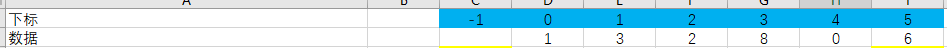2、我们不妨设最后一个元素，即a的值为基准值
3、我们在前面提到，快速排序(从小到大)的思想要求，在基准点左边的数都要比基准点小，右边的数都要比基准点大，因此我们需要两个指针，分别指向待排序数组的头尾。又因为数组的最后一个元素被我们用作基准点，所以直接从它的前一个元素开始比较即可，所以，我们采用先自减，再比较，即【--j】的方式。为了统一，我们同样在左边比较时，也采用【++i】的形式，因此，我们的两个指针begin和end在初始化时，应分别指向-1位置，和第5个位置。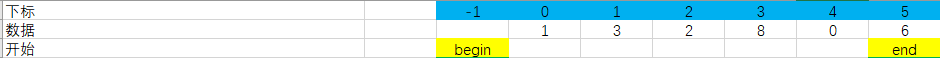4、结合在基准点左边数据要比基准点小，右边的要比基准点大的思想，我们可以很容易地写出一个大致的代码。因为我们不像冒泡排序那样能够知道整个排序过程要执行几次，因此采用死循环。

//快速排序的核心，返回基准点
int partition(Elemtype a[] , int l , int r)
{
//i，j为begin和end的初始位置
int i = l - 1;
int j = r;
//基准值
Elemtype x = a[r];
//因为不知要执行几次，所以使用死循环
while(1)
{
while(a[++i] < x);//假设数组已有序，那么，在基准值左边的所有元素的值<基准值
while(a[--j] > x);//假设数组已有序，那么，在基准值右边的所有元素的值>基准值
}
}
那么，接下来我们就根据这个大致的框架来分析一下什么时候结束循环，什么时候要交换数据，又是返回谁的下标作为基准点的问题。
5、我们根据算法框架，先进入了第一个while循环。我们知道，只有当数组中有比基准元素大于或者等于时，循环退出。在执行第一个循环时，既然是要把基准点左边排好序，所以应该是从头开始遍历。最好情况是在x左边的所有元素都比x来的小，当且仅当begin即i指针所指元素与基准元素x重合时，循环结束。根据本题，我们可以得到如下的一个排序过程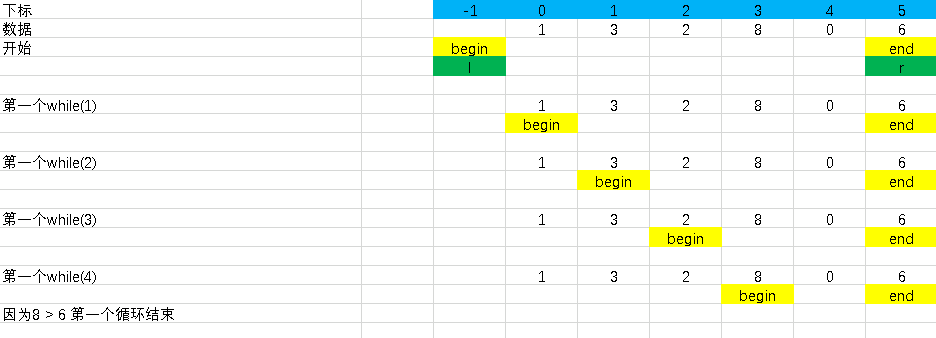由图可知，第一个循环能够自动退出。
6、退出第一个循环后，我们便进入了第二个循环。既然第一个循环是对数组左半边进行排序，那么第二个循环就是对数组的右半边进行排序了。依然以元素x为基准，根据前面的思想，数组右半边的元素应该都比x来的大。因此，我们不妨从数组右边开始往左遍历，只要end指针，即j指针所指的元素比x来的大就往前进，比x小就退出。所以，我们又能够得到如下的一个排序过程。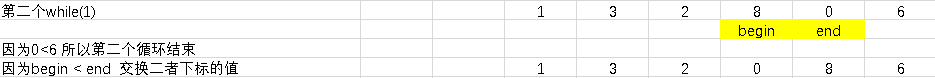7、第二个循环退出后，如果死循环未退出，在重新进入第一个循环之前，我们需要对下标为begin和end所指的元素进行交换，这一步非常重要。
假象一下，如果不进行交换，那么在下一次循环结束之后，begin会停留在6元素，end会停留在2元素，如下图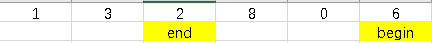无论说把6安置在begin或者end位置，都不能保证数组中以6为基准，左半边的元素比6小，右边的元素比6大了。
根据此时数组中的情况(交换值之后的数组)，我们会想，此时能不能将基准元素6交换到到数组中了呢？不难发现，如果把6交换在begin的位置，那么6之后的元素为8、0，不符合思想要求；如果把6交换到end的位置，我们发现在这个例子中是能保证以6为基准的数组中左半边的值比6小，右半边的值比6大的。但是考虑一下8 5 7 2 6 3这个情况。在第一次退出两个while循环后，begin与end的指针位置是这样的：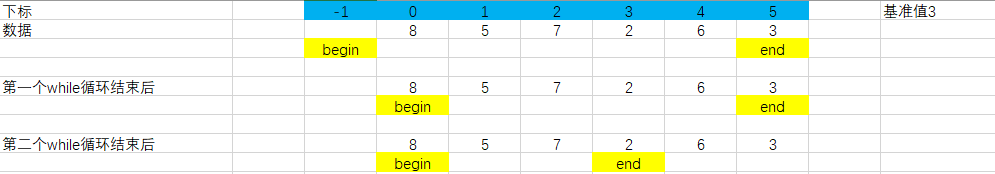可以发现，如果把3交换到end的位置，那么就完全错乱了，是吧~所以，我们退出死循环的条件构想得并不准确，
因此，我们并不能退出这个死循环。必须从第一个while循环重新开始。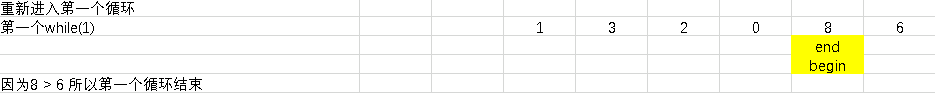8、第一个循环退出后，顺理成章进入第二个while循环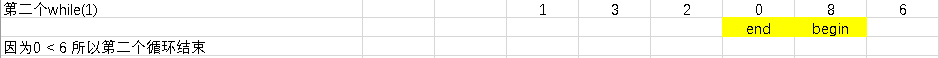9、此时，我们发现。只要把基准元素6与begin指针所指的元素8进行交换，刚好以6为基准的数组左边的所有元素都比6来的小，右边的元素都比6来的大。我们终于找到了基准元素的交换位置。找到交换位置后，我们就不需要再进行死循环了，因此，死循环退出的条件为end > begin，对应到程序中，就是i > j。我们的程序完善如下：

//快速排序的核心，返回基准点
int partition(Elemtype a[] , int l , int r)
{
//i，j为begin和end的初始位置
int i = l - 1;
int j = r;
//基准值
Elemtype x = a[r];
//因为不知要执行几次，所以使用死循环
while(1)
{
while(a[++i] < x);//假设数组已有序，那么，在基准值左边的所有元素的值<基准值

while(a[--j] > x);//假设数组已有序，那么，在基准值右边的所有元素的值>基准值

if(i > j)
{
break;
}
swap(&a[i],&a[j]);
}
}  

10、退出死循环之后，我们就找到了交换点，存于begin指针，即i指针中。我们只需要将基准元素6，与begin指针所指的元素8进行交换就行，即a[i] = a[r]，返回基准点

//快速排序的核心，返回基准点
int partition(Elemtype a[] , int l , int r)
{
//i，j为begin和end的初始位置
int i = l - 1;
int j = r;
//基准值
Elemtype x = a[r];
//因为不知要执行几次，所以使用死循环
while(1)
{
while(a[++i] < x);//假设数组已有序，那么，在基准值左边的所有元素的值<基准值

while(a[--j] > x);//假设数组已有序，那么，在基准值右边的所有元素的值>基准值

if(i > j)
{
break;
}
swap(&a[i],&a[j]);
}
swap(&a[i],&a[r]);
return i;
}

好了，经过以上10步，我们就能大致写好partition函数了。但是，这个函数并不健壮，还存在以下问题：
1、假设数组右边都已有序，即都比基准值大，那么第二个循环就无法退出了。
2、退出死循环的条件i能不能等于j 即 i==j 或begin == end
我们先解决第一个问题
如果数组右边的值都比x来的大，说明这个x是这个数组中最小的元素，应该放置在数组头，因此，假如我们从后向前遍历的指针end(即j)已经到达数组头(我们传入的begin，即l)时，就该退出循环。
我们再来解读第二个问题
如果i==j，即begin == end，说明起始指针已经与结束指针相遇。如果死循环继续进行，那么先执行的是第一个while循环，begin指针向右推进。那么问题来了，begin指针一旦向右推进，那么它所指元素的下标已经大于end了，这样再执行第二个循环已经没有意义了，等于说begin指针已经越到了end指针所管辖的范围。因此，当begin == end(即i == j)，就是基准元素x应该要插入的位置，应当退出死循环。
由此，我们知道，begin指针只管辖数组的左半边，end指针只管辖数组的右半边，而基准元素x，就是它们的“国界”。
所以，完整的partition函数如下

//快速排序的核心，返回基准点
int partition(Elemtype a[] , int l , int r)
{
//i，j为begin和end的初始位置
int i = l - 1;
int j = r;
//基准值
Elemtype x = a[r];
//因为不知要执行几次，所以使用死循环
while(1)
{
while(a[++i] < x);//假设数组已有序，那么，在基准值左边的所有元素的值<基准值

while(a[--j] > x){if(j == l) break;};//假设数组已有序，那么，在基准值右边的所有元素的值>基准值

if(i >= j)
{
break;
}
swap(&a[i],&a[j]);
}
swap(&a[i],&a[r]);
return i;
}

附上swap函数的定义

void swap(int *a , int *b)//把b的值给a，把a的值给b
{
int t = *a;
*a = *b;
*b = t;
}


最后是完整代码

#include <stdio.h>
//定义程序支持的数据类型
#define Elemtype int
void swap(int *a , int *b);
//快速排序的核心，返回基准点
int partition(Elemtype a[] , int l , int r);
//快速排序算法的递归实现 a为待排序数组 l为要开始排序的下标 r为要结束排序的数组下标 (l,r)就是排序范围
void quickSort(Elemtype a[] , int l , int r)
{
//基准位置
int i;
//递归出口
if(r <= l)
{
return;
}
//确定基准点
i = partition(a,l,r);
//基准点左边排序
quickSort(a,l,i-1);
//基准点右边排序
quickSort(a,i+1,r);
}

//快速排序的核心，返回基准点
int partition(Elemtype a[] , int l , int r)
{
//i，j为begin和end的初始位置
int i = l - 1;
int j = r;
//基准值
Elemtype x = a[r];
//因为不知要执行几次，所以使用死循环
while(1)
{
while(a[++i] < x);//假设数组已有序，那么，在基准值左边的所有元素的值<基准值

while(a[--j] > x){if(j == l) break;};//假设数组已有序，那么，在基准值右边的所有元素的值>基准值

if(i >= j)
{
break;
}
swap(&a[i],&a[j]);
}
swap(&a[i],&a[r]);
return i;
}

void swap(Elemtype *a , Elemtype *b)
{
//b的值交换到a , a的值交换到b
Elemtype t = *a;
*a = *b;
*b = t;
}

int main()
{
Elemtype a = {1,2,3,0,6,8};
int i = 0;
quickSort(a,0,5);
for(; i<6 ; i++)
{
printf("%d ",a[i]);
}
printf("\n");
return 0;
}
运行结果如下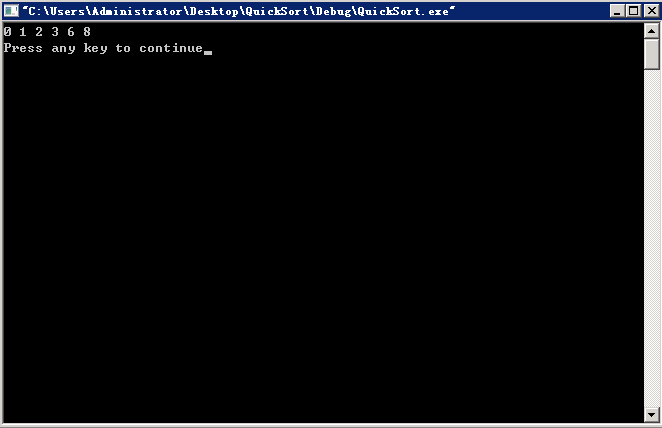最后简单说一下快速排序的时间复杂度为O(nlogn)
展开全文递归 快速排序
• 自定义一组有首地址为data的10个字的数组，请利用冒泡排序算法来编写程序，使该数组中的数按照从小到大的次序有序化。（注：10个字可以自己定义。） datas segment data1 dw 7,5,3,2,6,9,10,1,8 datas ends 冒泡...
汇编语言：冒泡排序算法 题目描述 自定义一组有首地址为data的10个字的数组，请利用冒泡排序算法来编写程序，使该数组中的数按照从小到大的次序有序化。（注：10个字可以自己定义。）
datas segment
data1 dw 7,5,3,2,6,9,10,1,8
datas ends

冒泡排序是一种较为简单的排序算法，需要使用嵌套循环。每一个外循环会将未排序数据中的最大值排到末尾，每一个小循环会将相邻两个数比较大小，从而使较大的数下沉，较小的数上浮。 本题中，我们需要使用条件转移指令，比较指令（CMP），交换指令（XCHG）。值得一提的是，CMP和XCHG的两个操作数不能同时为内存中的数据，但可以一个是寄存器，一个是内存数据。所以，在比较和交换数据的时候，我们需要将其中一个内存数据放到寄存器中。 （代码中有注释，可直接看代码）
思路： 将循环次数放入CX（设需要排序的数据有N个，则需要执行N-1个循环，即此时应MOV CX,9)。 1.CX-1判断CX是否符合循环条件，当CX=0时，程序结束；否则，SI置零，BX置2倍的CX作为小循环的判断条件（若数据使用DB定义则无需使用BX，可直接使用CX）； 2.将DATA1[SI]放入AX寄存器中，并与DATA1[SI+2]作比较（由于本体数据定义时DW，所以用+2，若使用DB定义数据则应+1），若小于等于，则执行第3步；否则交换DATA1[SI]和DATA1[SI+2]； 3.比较SI和BX，相等时执行第1步，否则，SI+2，跳转到第二步。 以上就是一个较为简单的冒泡排序法步骤，接下来看代码：
DATAS SEGMENT
DATA1 DW 7,5,3,2,6,9,10,1,8
DATAS ENDS

CODES SEGMENT
ASSUME CS:CODES,DS:DATAS
START:
MOV AX,DATAS
MOV DS,AX
MOV CX,9
L1: 					;最外层循环
MOV SI,0			;设置SI为零
CMP CX,0			;判断循环是否结束
JE EXIT
DEC CX				;cx-1
MOV BX,CX
;若数据以字节定义，则只需要将bx置为cx即可
L2:
MOV AX,DATA1[SI]	;不能直接比较内存中的数字，所以我们需要将其中一个数字放到寄存器AX中
CMP AX,DATA1[SI+2]	;比较两个数
JLE L3				;小于等于的话，则直接跳到下一对数据的比较
XCHG AX,DATA1[SI+2]	;若大于，则通过两个XCHG语句，交换两内存中的数字
XCHG AX,[SI]
;内层循环结束时跳到外层循环
L3:
CMP SI,BX
JE L1
JMP L2
EXIT:
MOV AH,4CH
INT 21H
CODES ENDS
END START

排序结果 冒泡排序前运行前内存数据内容：冒泡排序后：冒泡排序运行正确，此题目完成！
展开全文• 自定义一组有首地址为data的10个字的数组，请利用冒泡排序算法来编写程序，以使该数组中的数按照从小到大的次序有序化。（注：10个字可以自己定义。） datas segment buffer dw 7,5,3,2,6,9,10,1,8 datas ends ...编程语言
•零基础 动态规划
• ## 排列组合算法

千次阅读 2016-10-21 13:45:41
排列和组合是两种不同的算法排列所求的k个数，数的排序不同则为种不同情况，总共有k!中可能情况求全排列最常用的算法就是按照字典式排序(STL)，字典式排序从第位开始找最小的数，然后依次找每位能够存在的...组合 STL C++
• 主要功能：用选择排序按从小到大的顺序排列数 #include &amp;amp;lt;stdio.h&amp;amp;gt; #define N 10 void SelectionSort(int *a,int n) { int i,j,temp; for(i=0;i&amp;amp;lt;n-1;i++) { for(j=...C语言 选择排序源码
• 有N个正实数(注意是实数，大小升序排列) x1 , x2 ... xN，另有个实数M。 需要选出若干个x，使这几个x的和与 M 最接近。 请描述实现算法，并指出算法复杂度。 #define M 8 #define N 20 int minDif = INT_MAX; ...
• A B F C D E 分析:在程序中引入变量 a, b, c, d, e, f, 并让它们分别顺序取 1 至 6 的整数, 在它们互不相同的条件下,测试由它们排成的三角形边上的变量之和是否相等,如相等即为种要求的排雷,把它们全排列
• * 通过冒泡排序实现从小到大排列 * * 冒泡排序：将数组中第位与第二位比较，小的数字放在前面，然后再由第二位与第三位作比较，小的数放前面； * 按照这个顺序，得出最大的那个数，并且这个最大的数放在最...
• 类似于气泡上升的动作，会将数据在数组中从大到小或者从小到大不断地向前移动。 这个算法的名字由来是因为越小的元素会经由交换慢慢“浮”到数列的顶端（升序或降序排列），就如同碳酸饮料中二氧化碳的气泡最终会...shell linux
• 重点思路：将xy捆绑到一块排序，因为y是倒叙需要计算下对应正序时的值，也就是拿y最大值减去当前值，得到x升序y升序组合数xy，需要考虑y位数问题 import java.util.Arrays; public class Test { public ...
• 排列组合算法 我们都知道排列与组合的个数可以利用公式很容易的求出来，但是要是把这些排列组合的序列一一输出怎么办呢？ 下面结合《组合数学》（第四版）卢开澄卢华明编著，好好总结排列与组合的算法排列...
• 给出一组关键字序列{29,18,25,47,58,12,51,10}，分别给出用希尔排序、直接选择排序算法从小到大排序的结果。 参考施老师等编著的《数据结构》，本代码参考了赵同学的报告。 算法思想： 选择排序的基本思想是依次从待...快速排序
• 　给定个长度为n的数列，将这个数列按从小到大的顺序排列。1<=n<=200 输入格式 　第行为个整数n。 　第二行包含n个整数，为待排序的数，每个整数的绝对值小于10000。 输出格式 　输出行，按从小到大的...
• 上图是按从小到大的顺序排列，从大到小以此类推。 import java.util.Scanner; public class Prog35 { public static void main(String[] args) { System.out.print("请输入一组数："); Scanner s...
• 今天小编就为大家分享篇用python实现将数组元素按从小到大的顺序排列方法，具有很好的参考价值，希望对大家有所帮助。一起跟随小编过来看看吧
• 输出：[123(1) , 132(2) , 213(3) , 231(4) , 312(5) , 321(6)]—>X=2 首先看到题目想到的是生成个从少到大的全排列的数组，然后再遍历数得到对应的序号(数组下标加1)，又或者想到个个从小到大的生成push进...
•  本程序的思路是开个数组，其下标表示1m个数，数组元素的值为1表示其下标  代表的数被选中，为0则没选中。  首先初始化，将数组前n个元素置1，表示第个组合为前n个数。  然后从左右扫
• 数据结构：是指相互之间存在种或多种特定关系的数据元素的集合用计算机存储、组织数据的方式。数据结构分别为逻辑结构、（存储）物理结构和数据的运算三个部分。 为什么要学数据结构？ 首先，因为数据结构作为...新手 教程 C语言
• 编写个将A和B中所有元素结点组成个新的从小到大的有序顺序表C的算法，要求所有重复元素只保留个。 分析 还是在归并算法上进行修改。 代码 核心代码： /* 归并A和B表 */ /* A[]指的是A顺序表；An指的是A...
• 　首先看到题目想到的是生成个从少到大的全排列的数组，然后再遍历数得到对应的序号(数组下标加1)，又或者想到个个从小到大的生成push进数组，然后判断该数是不是当前题目给的数，如果是的话要求的序号就是...
• 例如，由1,2,3组成的所有排列从小到大的依次为： 123,132,213,231,312,321 由1,2,3,4组成的所有排列： 1234, 1243, 1324, 1342, 1423, 1432, 2134, 2143, 2314, 2341, 2413, 2431, 3124, 3142, 3214, 3241, ...
• 小根堆实现从小到到排序： 每次删除并输出（直接输出或存放于数组）顶部元素， 维护此时的小根堆，反复上行操作，直到堆为空为止 #include <bits/stdc++.h> using namespace std; int h,n; void ...• 大到小： #include using namespace std; int main() {  int a; int n; cin>>n; for(int i=0;i { cin>>a[i]; } for(int i=0;i { for(int j=0;j { if(a[j] swap(a[j],a[j+1]); } } for(int i...